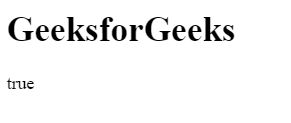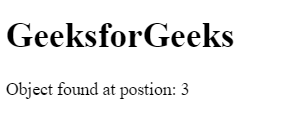# How to check if an array includes an object in JavaScript?

There are various methods to check an array includes an object or not.

Using includes() Method: If array contains an object/element can be determined by using includes() method. This method returns true if the array contains the object/element else return false.

Syntax:

`array.includes( element/object, startingPosition )`

Example:

 ` ` `<``html``> ` ` `  `<``head``> ` `    ``<``title``> ` `        ``JavaScript includes() Method ` `    `` ` ` ` ` `  `<``body``> ` ` `  `    ``<``h1``>GeeksforGeeks ` `     `  `    ``<``p` `id``=``"geeks"``> ` `     `  `    ``` `    ``<``script``> ` `        ``var obj = {"geeks1":10, "geeks2":12} ` `        ``var gfg = ["geeks1", "geeks2", obj]; ` `         `  `        ``/* Use JavaScript includes() method */ ` `        ``var num = gfg.includes(obj, 0); ` `         `  `        ``document.getElementById("geeks").innerHTML ` `                    ``= num; ` `    `` ` ` ` ` `  `         `

Output:Using some() Method: The some() method uses the function for its evaluation and it executes the function once for each element present in the array. If it finds the object/element in the array then it returns true and stop the execution for remaining elements otherwise return false.

Syntax:

`array.some( function(currValue, arrIndex, arrObj), this )`

Example:

 ` ` `<``html``> ` ` `  `<``head``> ` `    ``<``title``> ` `        ``JavaScript includes() Method ` `    `` ` ` ` ` `  `<``body``> ` ` `  `    ``<``h1``>GeeksforGeeks ` `     `  `    ``` `    ``<``script``> ` `     `  `        ``/* Declare an array */ ` `        ``var arr = ["geeks1", "geeks2", "geeks3", ` `                    ``{1:"geeks4", 2:"geeks5"}]; ` `                     `  `        ``var boolVar = arr.some(  ` `            ``value => { return typeof value == "object" } ); ` `             `  `        ``document.write(boolVar); ` `    `` ` ` ` ` `  `                     `

Output:Using filter() Method: The filter() method creates the array of all those elements/objects that passes the checking condition.

Syntax:

`array.filter( function(currValue, arrIndex, arrObj), this )`

Example:

 ` ` `<``html``> ` ` `  `<``head``> ` `    ``<``title``> ` `        ``JavaScript includes() Method ` `    `` ` ` ` ` `  `<``body``> ` ` `  `    ``<``h1``>GeeksforGeeks ` `     `  `    ``<``p` `id``=``"geeks"``> ` `     `  `    ``` `    ``<``script``> ` `        ``var obj = {"geeks1":10, "geeks2":12} ` `        ``var arr = ["geeks1", "geeks2", "geeks3", obj]; ` `         `  `        ``if(arr.filter(value=> value==obj).length > 0) ` `            ``document.write("true"); ` `        ``else ` `            ``document.write("false"); ` `    `` ` ` ` ` `  `                     `

Output:Using findIndex() Method: The findIndex() method returns the position of searched object/element if it present in the array and stop the execution for rest elements. If the element/object not found then return -1.

Syntax:

`array.findIndex( function(currValue, arrIndex, arrObj), this )`

Example:

 ` ` `<``html``> ` ` `  `<``head``> ` `    ``<``title``> ` `        ``JavaScript includes() Method ` `    `` ` ` ` ` `  `<``body``> ` ` `  `    ``<``h1``>GeeksforGeeks ` `     `  `    ``` `    ``<``script``> ` `     `  `        ``/* Declare an array */ ` `        ``var arr = ["geeks1", "geeks2", "geeks3", ` `                ``{"geeks1":10, "geeks2":12}]; ` `                 `  `        ``var num = arr.findIndex(  ` `            ``value => { return typeof value == "object" } ); ` `             `  `        ``document.write("Object found at postion: " + num); ` `    `` ` ` ` ` `  `                     `

Output:My Personal Notes arrow_drop_upCheck out this Author's contributed articles.

If you like GeeksforGeeks and would like to contribute, you can also write an article using contribute.geeksforgeeks.org or mail your article to contribute@geeksforgeeks.org. See your article appearing on the GeeksforGeeks main page and help other Geeks.

Please Improve this article if you find anything incorrect by clicking on the "Improve Article" button below.

Article Tags :

Be the First to upvote.

Please write to us at contribute@geeksforgeeks.org to report any issue with the above content.